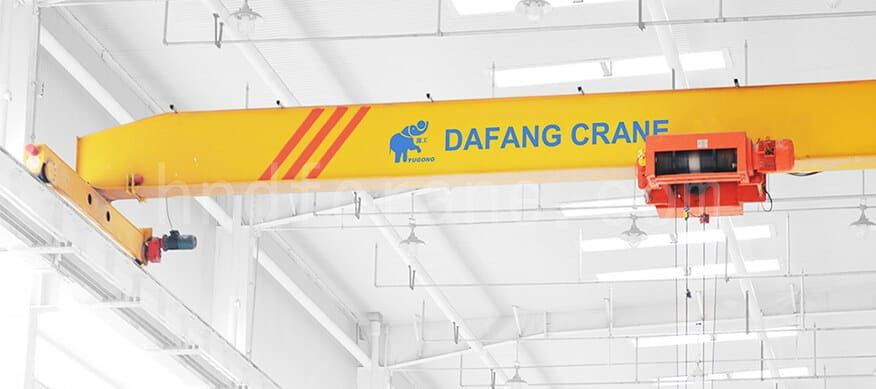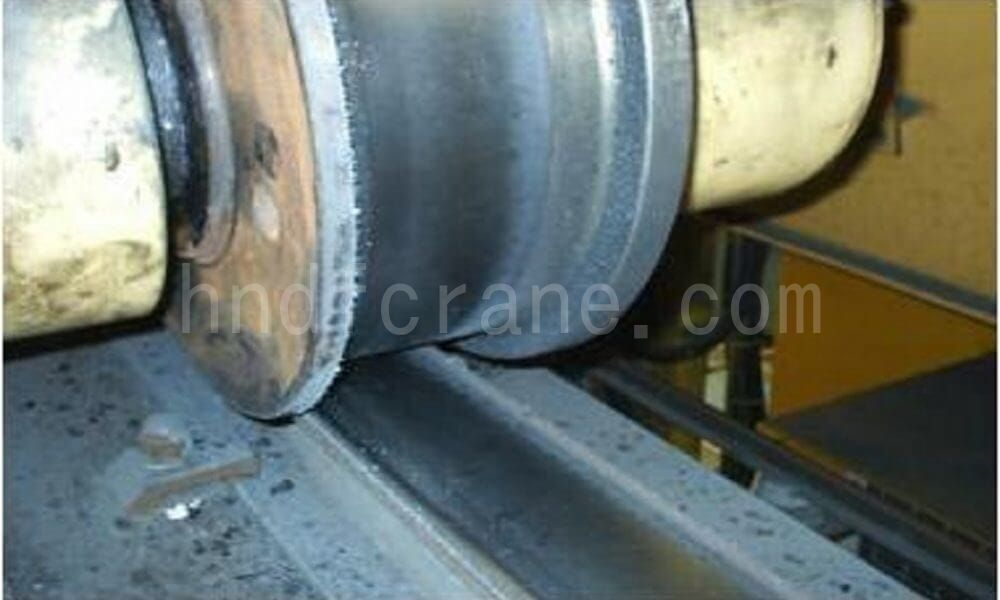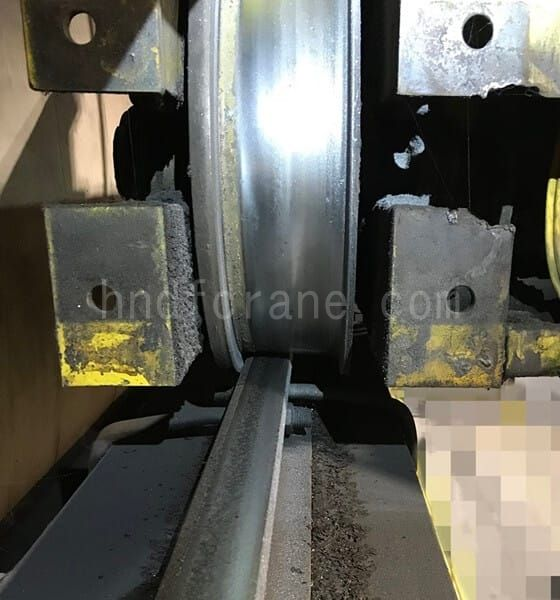August 07, 2021The wheel pressure load of the overhead crane is the vertical pressure of the wheel on the track. Crane operating mechanism parts and metal structure strength calculation mainly depends on the maximum wheel pressure load of the crane, while it also provides a basis for the design of wheel devices, but also for the design of the track support structure provides the original data. And the minimum wheel pressure load is mainly used for running mechanism starting and braking wheel slip test.The calculation of the wheel pressure load of the overhead crane, that is, the calculation of the total pressure of the pivot point. The calculation of wheel pressure load is divided into the calculation of the wheel pressure load under the moving load and the calculation of the wheel pressure load under the super static structure.### Calculation of wheel pressure load under super static structure

The four-point supporting structure of the overhead crane is super static, the distribution of the supporting reaction force is not only related to the load, but also related to the structural rigidity of the frame, the foundation rigidity, the manufacturing and installation accuracy of the frame structure, and the elasticity and flatness of the track, etc. However, to calculate the impact of these factors on the supporting reaction force is quite time-consuming, and it is difficult to estimate the unevenness of the track. Therefore, the calculation of wheel pressure load under super-stationary structure generally adopts the approximate solution method, and the error difference between the approximate solution method and the exact solution method has not been studied yet.

### Example analysis:

First,known: lifting capacity: Q = 20 tons, span: L = 22.5 m, the number of wheels: 4, the total weight of the crane (including the trolley) total = 32.5 tons.

Trolley weight: G = 7.5 tons, spreader weight: 0.5 tons, the minimum distance from the hook centerline to the end beam centerline L1 = 1.5 meters (large hook limit position)

Second, the calculation process

So the maximum wheel pressure load Pmax=19317, the minimum wheel pressure load Pmin=6517kg